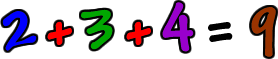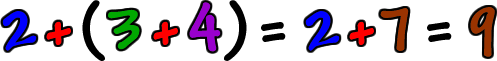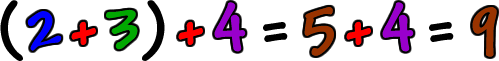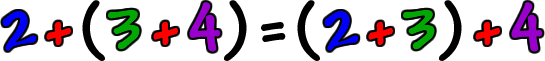Yeah, that's an easy one!What if we grouped the numbers...  Put the 3 and the 4 in parenthesis?  Remember the Order of Operations ...  In math, we always do what's in the parenthesis first!  So, if we do this, will we get the same thing?Yep!

Now, what if we group the numbers differently...  Will we get the same answer again?Remember that, if both things equal 9, then those things have to equal each other!